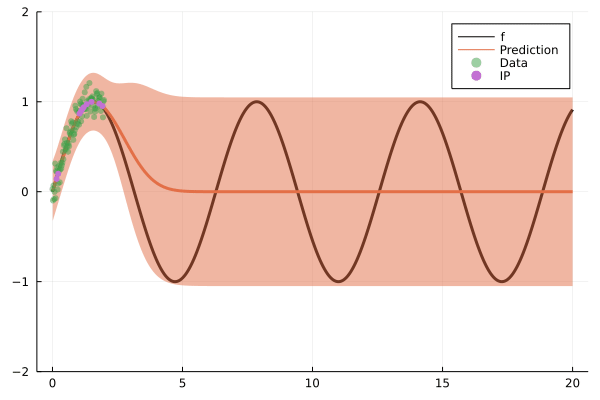# Online Gaussian Process## Preliminary steps

using Plots
using AugmentedGaussianProcesses
using MLDataUtils, Distributions

### We create a toy dataset with a noisy sinus

N = 2000
σ = 0.1
X, y = noisy_sin(N, 0, 20; noise=σ)
X_train = X[1:2:end];
y_train = y[1:2:end]; # We split the data equally
X_test = X[2:2:end];
y_test = y[2:2:end];
scatter(X_train, y_train)

### Plot model at each step

function plot_model(model, X, X_test, X_train, y_train)
y_pred, sig_pred = proba_y(model, X_test)
plot(X, sin; lab="f", color=:black, lw=3.0, ylims=(-2, 2))
plot!(X_test, y_pred; ribbon=sqrt.(sig_pred), lab="Prediction", lw=3.0)
scatter!(X_train, y_train; msw=0.0, alpha=0.5, lab="Data")
return scatter!(first.(model.Z), mean(model); lab="IP")
end
plot_model (generic function with 1 method)

## Model training

### Create a kernel

k = SqExponentialKernel();

### Create an inducing point selection method

IP_alg = OIPS(0.8);

### Create the model and stream the data

model = OnlineSVGP(k, GaussianLikelihood(σ), AnalyticVI(), IP_alg; optimiser=false)
anim = Animation()
size_batch = 100
let state = nothing
for (i, (X_batch, y_batch)) in
enumerate(eachbatch((X_train, y_train); obsdim=1, size=size_batch))
_, state = train!(model, X_batch, y_batch, state; iterations=5)
plot_model(
model, X, X_test, X_train[1:(i * size_batch)], y_train[1:(i * size_batch)]
)
frame(anim)
end
end
gif(anim; fps=4)This works just as well with any likelihood! Just try it out!